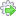How to use ET GeoWizards as a stand alone application

A. Via the User Interface

1. Clicking on the ET GeoWizards 12 will introduce the ET GeoWizards main dialog
2. Select the appropriate group of functions in the navigation panel on the left.
3. Select the function required.
4. The appropriate topic of the User Guide will be displayed in the Help Window
5. To run the selected function click the GO button or the Run iconnext the the function name. You can also double click the function name.

Note:

The dialog for each function has a Help Tab that contains the full help topic for the current function.

B. In Python scripts.

All the functions of ET GeoWizards can be used in Python Scripts by calling ETGWRun.exe located in the installation folder of ET GeoWizards using the Subprocess Python module. ET GeoWizards 12 is a native 64-bit application, but since it runs in a separate process, it can be used from 32 and 64 bit Python.

Below is an example of using a function of ET GeoWizards within a Python script

 Calling the script RotateShapes from Command Prompt python.exe RotateShapes.py "c:\00\aaaa.shp" "c:\00\aaaaRotated.shp" "45" "" "5" "5" import sys, subprocessargList = sys.argvprint len(argList)if len(sys.argv) < 5:     print "Usage:" , "RotateShapes {origin_point_dataset} {Origin_X} {Origin_Y}" else:     etgwPath = r"C:\Program Files\ETSpatial Techniques\ETGeo Wizards\ETGWRun.exe"     etFunction = "RotateShapes"     inputDataset = argList     outputDataset = argList     rotation_angle = argList     origin_point_dataset = argList     Origin_X = "0"     Origin_Y = "0"     if len(sys.argv) >=6:          Origin_X = argList     if len(sys.argv) >=7:          Origin_Y = argList     print "Input: " , inputDataset     print "Output: " , outputDataset      retCode = subprocess.call([etgwPath, etFunction, inputDataset, outputDataset, rotation_angle, origin_point_dataset, Origin_X,Origin_Y])     if retCode == 0:          print "Success"     else:     print retCode The location of ETGWRun.exe can be derived from the registry using:hKey = _winreg.OpenKey (_winreg.HKEY_LOCAL_MACHINE, r"SOFTWARE\ETSpatial Techniques\ETGeo Wizards", 0, _winreg.KEY_READ|_winreg. KEY_WOW64_64KEY) myPath = _winreg.QueryValueEx(hKey, "InstallPath")etgwPath = myPath+r"ETGWRun.exe"_winreg.CloseKey(hKey)

C. In .NET applications

The functionality of ET GeoWizards can be also used in custom .NET applications. See this topic for details

D. From the Command Prompt

The functionality of ET GeoWizards can be executed directly from the DOS command prompt. Example:

ETGWRun.exe "RotateShapes" "c:\00\aaaa.shp" "c:\00\aaaaRotated.shp" "45" "" "5" "5"

Notes:

• Please read the topic for a specific function for parameters description and syntax